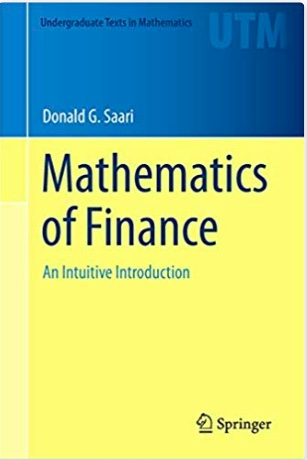# Math 176: Math of Finance (English)

## Course Information

After reviewing tools from probability, statistics, and elementary differential and partial differential equations, concepts such as hedging, arbitrage, Puts, Calls, the design of portfolios, the derivation and solution of the Blac-Scholes, and other equations are discussed.

The notes for this course, Math 176, Mathematics of Finance, have been published as a book. See below for complete information.

Mathematics Dept. | Physical Sciences Sch. | University of California, Irvine
Keywords: Math,Saari,Finance,probability and statistics,differetial equations,partial differential equations,hedging,arbitrage,Puts and Calls,portfolio design,Blac-Sholes
The book(s) listed below are provided only as a service to learners who are looking for further exploration of this topic by the listed professor or speaker. Unless otherwise stated, they are not licensed under a Creative Commons license.

Books used in this class:Title:
Mathematics of Finance: An Intuitive Introduction
Author:
ISBN:Author:
Donald Saari Ph.D.
Title:
Professor
Department:
Mathematics